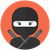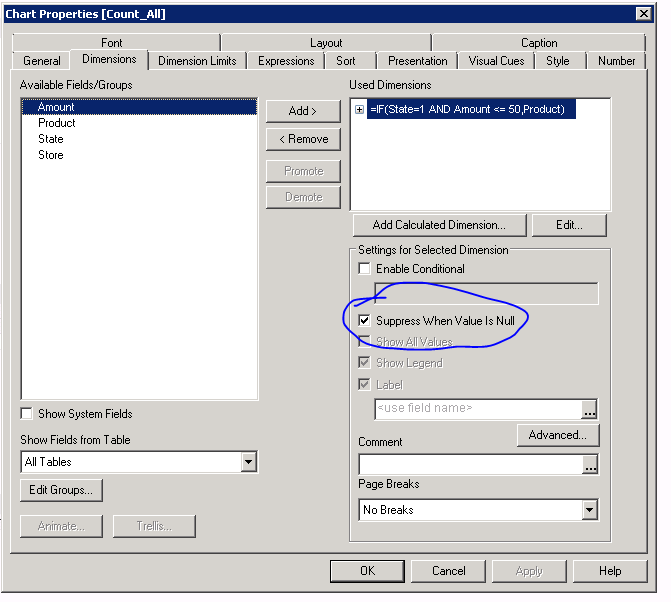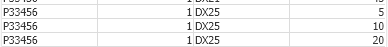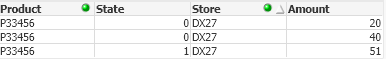# QlikView App Dev

Discussion Board for collaboration related to QlikView App Development.

Announcements
QlikWorld 2023, a live, in-person thrill ride. Save \$300 before February 6: REGISTER NOW!
cancel
Showing results for
Did you mean:Contributor III

## Sum/count if amount within store is under 50

Hi,

I am having some issues with getting the right results in some calculations. I think I need to use the aggr function, but I’m doing something wrong.

I want to count and sum data based on some criteria.

I have the following fields:

• Product
• Amount
• Store
• State

If the status is 0 they always need to be included.

However when it gets more advanced I get issues.

I want to do this:

If status is 1 and the amount per store is under 50 I want to include the rows. If they however are 50 or above, I do not want them to be a part of my result.

I have tried this:

count(distinct if(State =1 and Aggr(sum(AMOUNT),Store)<50,count(AMOUNT),'0'))

… gives me nothing

if(State=1 and Aggr(sum(AMOUNT),Store)<50,count(AMOUNT),'0')

… gives me 0

I have also tries without the State field

count(distinct aggr(if(sum(AMOUNT)<50,store),AMOUNT))

… gives me 0

Since I can’t share my data, I have created a sample file, that illustrated my issue.

What I would want as result in the sample:

Sum:

Always sum if state 0: 110

Only sum state one, when the amount within each store is under 50. Should be: 45

Total sum wanted: 155

Count:

Always count if state 0: 4

Only count state one, when the amount within each store is under 50. Should be: 4

Total count wanted: 8

It seems like I limit my result to only one row pr. store. I guess that makes sense, but I have no idea on how to achieve what I want.

Any guru’s that can help me with this one?

Labels (4)

• ### sum

1 Solution

Accepted SolutionsMVP

Try this:

=Sum({<State={0}> + <State={1},Store={"=Sum({<State={1}>}Amount)<50"}>}Amount)

=Count({<State={0}> + <State={1},Store={"=Sum({<State={1}>}Amount)<50"}>}Amount)

8 RepliesContributor III

Hello Zwax,

If I understand your requirement correctly, just change your dimension to below.

=IF(State=1 AND Amount <= 50,Product)

Find attached.

Thanks.

JeffContributor III

Just to add on, remember to tick 'Suppress When Value is Null' in order to hide the amount greater than 50.Contributor III

Change the dimension to below instead. (Changed from <= 50 to <50)

=IF(State=1 AND Amount < 50,Product)MVP

Your sample data and explanation don't look to be in sync. Could you go through your sample app and confirm your expected output?Contributor III
Author

Hi,

It's not giving me the results I want. I probably didn't explain it good enough - sorry 🙁

When I wrote “..and the amount per store is under 50..” I meant the total amount within the store. Or in other words: if the state is 1 and the sum of the amount within the same store is under x.

Example:

Store DX21..should not be summed and counted since the sum of the amounts are 55 and therefore over 49.

Store DX25..should be summed and counted since the summed amount is under 50.

Store DX27.. should be counted and summed by the 2 first rows since they have state 0 (state 0 should always be counted and summed). The third row should however not be counted or summed since it’s above 50.

Actually thinking about it, there is probably even 2 dimensions. Lets say the last number (store DX27) was 45, I would want it to be a part of the result. The total sum of the store is 50 or above, but not in within state 1.

Hope that explains what I’m trying to achieve. Did it make sense?MVP

Try this:

=Sum({<State={0}> + <State={1},Store={"=Sum({<State={1}>}Amount)<50"}>}Amount)

=Count({<State={0}> + <State={1},Store={"=Sum({<State={1}>}Amount)<50"}>}Amount)Contributor III

Hi, find attached.

Included the Dimension and using Ifelse statement in expression.

Thanks.Contributor III
Author

@tresesco wrote:

Try this:

=Sum({<State={0}> + <State={1},Store={"=Sum({<State={1}>}Amount)<50"}>}Amount)

=Count({<State={0}> + <State={1},Store={"=Sum({<State={1}>}Amount)<50"}>}Amount)

Amazing solution 😊 Thank you.

I really need to read up on set analysis...Community Browser